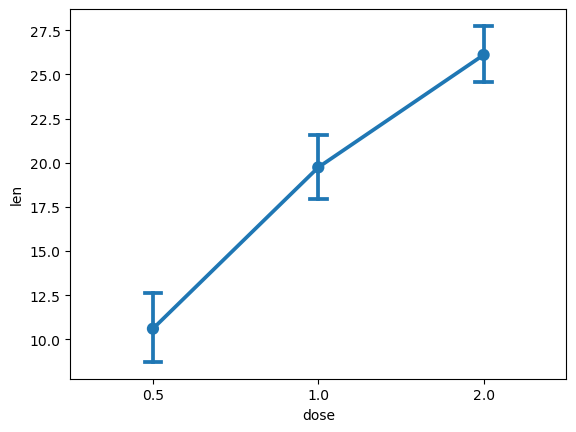# How to analyze the sample means of different treatment conditions

## Description

In a single-factor experiment with three or more treatment levels, how can we compare them to see which one impacts the outcome variable the most?

## Using Matplotlib and Seaborn, in Python

View this solution alone.

The solution below uses an example dataset about the teeth of 10 guinea pigs at three Vitamin C dosage levels (in mg) with two delivery methods (orange juice vs. ascorbic acid). (See how to quickly load some sample data.)

1
2
from rdatasets import data
df = data('ToothGrowth')


To visually plot the means of the length of the tooth based on the Vitamin C dosage levels we can create a pointplot. We will have to import the seaborn and matplotlib.pyplot packages to be able to create it.

1
2
3
4
5
6
import seaborn as sns
import matplotlib.pyplot as plt
sns.pointplot( x = 'dose', y = 'len', data = df,
ci = 95,         # ci stands for Confidence Interval
capsize = 0.1 )  # the width of the "caps" on error bars
plt.show()

1
2
3
4
5
/tmp/ipykernel_4429/664134235.py:3: FutureWarning:

The ci parameter is deprecated. Use errorbar=('ci', 95) for the same effect.

sns.pointplot( x = 'dose', y = 'len', data = df,The point plot informs us that as the dosage levels increase, the tooth length also increases.

To obtain the actual numbers, we can use the groupby function to compute the treatment level means, and the mean function to compute the mean for the entire column.

1
df.groupby('dose')['len'].mean()

1
2
3
4
5
dose
0.5    10.605
1.0    19.735
2.0    26.100
Name: len, dtype: float64

1
df['len'].mean()

1
18.813333333333336


If you wish to display the difference between the overall mean and the group means, you can subtract the overall mean from the treatment level means.

1
df.groupby('dose')['len'].mean() - df['len'].mean()

1
2
3
4
5
dose
0.5   -8.208333
1.0    0.921667
2.0    7.286667
Name: len, dtype: float64


See a problem? Tell us or edit the source.

## Using gplots and emmeans, in R

View this solution alone.

The solution below uses an example dataset about the teeth of 10 guinea pigs at three Vitamin C dosage levels (in mg) with two delivery methods (orange juice vs. ascorbic acid). (See how to quickly load some sample data.)

1
df <- ToothGrowth


To visually plot the means of the length of the tooth based on the Vitamin C dosage levels we can create a pointplot. We will use the gplots package. In the code below, bars=TRUE gives 95% confidence intervals for the means.

1
2
3
# install.packages("gplots") # If you have not yet installed it
library(gplots)
plotmeans(len~dose, data=df, bars=TRUE)

1
2
3
4
5
6
Attaching package: ‘gplots’

The following object is masked from ‘package:stats’:

lowess


The point plot informs us that as the dosage levels increase, the tooth length also increases.

To obtain the actual numbers, we can use the code below. The first line converts the numerical dosage values to a categorical variable, which may not be necessary if your data was already categorical.

1
2
3
df$dose.factor = as.factor(df$dose)
aov1 = aov(len~dose.factor, data=df)
model.tables(aov1, type='means')

1
2
3
4
5
6
7
8
9
Tables of means
Grand mean

18.81333

dose.factor
dose.factor
0.5      1      2
10.605 19.735 26.100


If you wish to display the difference between the overall mean and the group means, you can simply omit the type='means' parameter.

1
model.tables(aov1)

1
2
3
4
5
6
Tables of effects

dose.factor
dose.factor
0.5      1      2
-8.208  0.922  7.287


To also see the specific values for the confidence intervals plotted earlier, we can use the emmeans package (Estimated Marginal Means or Least-Squares Means).

1
2
3
# install.packages("emmeans") # If you have not yet installed it
library(emmeans)
emmeans(aov1,'dose.factor')

1
2
3
4
5
6
dose.factor emmean    SE df lower.CL upper.CL
0.5           10.6 0.949 57     8.71     12.5
1             19.7 0.949 57    17.84     21.6
2             26.1 0.949 57    24.20     28.0

Confidence level used: 0.95